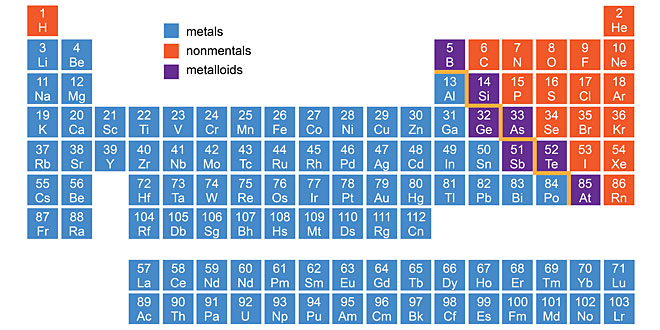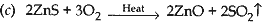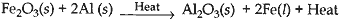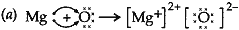Monday , May 25 2020# Metals and Non-metals: 10th Science

#### Question: List three properties of sodium in which it differs the general physical properties of most metals.

1. Sodium is so soft that it can be cut with a knife.
2. It has low density.
3. It has low melting point.

#### Question: Describe ionic compound on the basis of the following properties: (1). Strong force of attraction between positive and negative ions. (2). Solubility of compounds in water. (3). Electrical conductivity.

1. Due to strong force of attraction between the positive and negative ions, ionic compounds are solids. These compound are generally brittle and break into pieces when pressure is applied.
2. Ionic compounds are generally soluble in water.
3. Ionic compounds in the solid state do not conduct electricity but aqueous and molten state they do conduct electricity.

1. Mercury
2. Aluminium
3. Basic

#### Question: (1) ‘Sodium is a highly reactive metal and it cannot be obtained from its oxide by heating with carbon’. Give reason. (2). How can sodium be obtained from sodium chloride?

1. Sodium cannot be obtained from its oxide by heating with carbon because carbon cannot reduce the oxides of sodium.
2. Sodium can be obtained from sodium chloride by the process of electrolytic reduction.

#### Question: (1) Define the term ‘anode mud’. Name the electrode made of pure metal. (2) Give the reactions taking place at cathode and at anode during the electrolytic refining of copper.

1. The insoluble impurities which settle down at the bottom of the anode are known as anode mud.
Cathode is made of pure metal.
2. At anode: Cu(s) → Cu2+(aq)   +   2e
At cathode: Cu2+(aq)   +   2e–  → Cu(s)

#### Question: Suggest the method of reduction for the following metals during their metallurgical metals during their metallurgical processes. (1) Metal ‘A’ which is one of the last second or third position in reactivity series. (2) Metal ‘B’ which gives vigorous reaction even with water and air. (3) Metal ‘C’ which is kept in middle of reactivity series.

1. By the process of heating.
2. By heating with carbon.
3. By the process of roasting and calcination.

#### Question: Name two metals which are purified by electrolytic refining. Mention the anode, cathode and the electrolytic used in the refining process. At which electrode would the pure metal be deposited?

1. Copper, zinc
2. Impure metal is made the anode and thin strip of pure metal is made the used as an electrolyte.
3. Pure metal from the electrolyte is deposited on the cathode.

#### Question: balanced equation in each case: (a) Magnesium is treated with very dilute HNO3. (b) Aluminium powder is added to Fe2O3. (c) Zinc sulphide is roasted.

(a) Mg + 2HNO3  →  Mg(NO3)2 + H2
(b)Fe2O3  +  2Al → 2Fe + Al2O3 + Heat#### Question: (a) Consider the following chemical equation: Fe2O3  +  2Al → Al2O3 + 2Fe Name two categories in which you can place this reaction. (b) Define the term ‘Oxidation’ of a substance and name the substance oxidised and the oxidising agent in the above reaction.

1. (i) Thermite reaction, (ii) Redox reaction.
2. Oxidation means addition of oxygen to a substance or removal of hydrogen from a substance.
Aluminium is oxidised.
Fe2O3  is oxidising agent.

#### Question: What is thermit reaction? How is it used to join the railway tracks or cracked machine pats?

Answer: The reaction which is highly exothermic and in which metal is produced in molten state is known as thermit reaction. Consider the following displacement reaction:In this reaction, when iron (III) oxide (Fe2O3) is heated with aluminium powder (Al), the amount of heat evolved is so large that the metal iron (Fe) is produced in molten state. Hence, this reaction is used to join railway tracks or cracked machine part.

#### Question: (a) Show the formation of MgO by the transfer of electrons. (b) Name the cation and anion. (c) Write three properties of ionic compounds.(b) (i) Cation is positively charged [Mg2+] (ii) Action is negatively charged (O2-).
(c) A solution of ionic compounds contain ions since electricity conduction requires movement of charged particles they conduct electricity.
(i) Soluble in water.
(ii) Good conductor of electricity in molten state and aqueous solution.
(iii) High melting and boiling point.

## Garbage In Garbage Out: 6th CBSE NCERT Science Ch 16

Garbage In Garbage Out – 6th Class NCERT CBSE Science Chapter 16 Question: Distinguish between bio-degradable and …